The best tools to make your project dreams come true

Login or Signup
﻿

# Circuit Python TV Zapper with Circuit Playground Express

###Introduction

Courtesy of Adafruit

Pew Pew! Televisions are toast when you build this project, a universal TV zapper made with just your Circuit Playground Express. This guide will not only show you how to make a DIY universal remote, we'll also show how to grab data using a logic analyzer, parse it with Jupyter notebooks, compress it to fit into our little CircuitPython boards, and take advantage of the Python eval function to dynamically load data into memory.

BUT FIRST...a story...

When I first made the Circuit Playground (a.k.a the 'Classic' AVR based one) I showed it off to my inspirational friend Mitch Altman. Mitch is a wonderful maker who travels to events and maker spaces to teach people soldering and electronics. We met over a decade ago when our mutual friend pt suggested we work together on the TV-B-Gone kit. See, Mitch had been making and selling the TV-B-Gone, which looked like this:And that one LED could reach pretty darn far, maybe 50 feet. But we wanted to see if we could design one that would go 300 feet! So we worked on a kit version and made this: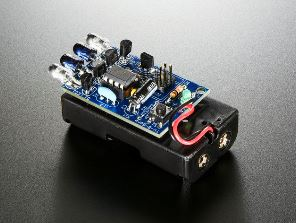TV-B-Gone Kit

When we were putting together the TV-B-Gone kit, we started imagining a dystopian future, filled with televisions, where this kit would be super useful and we'd be a hero...

With a total of four high powered LED blasters. It worked great, and Mitch, as we said, goes to events and does workshops, so this was a popular workshop kit.

But back to the tale at hand, I showed him the Circuit Playground and he said that it was really neat but if it had an IR LED it could act like a TV-B-Gone! And, frankly, I agreed. I couldn't fit an IR LED onto the original, but when I designed the Express version, I made some space for an IR LED and receiver. So, here we are!

In this guide, we'll build a TV zapper using just your Circuit Playground Express, a battery pack, and CircuitPython. We also have a bonus page for making a miniature zapper using a Gemma M0

You can use any CircuitPython board to make this project, but since the CPX has infrared built in, we're going to use that as the example hardware.

Grabbing Data

Before we begin, we need to get some data. In particular, we'll need the 'power' codes for each TV we want to disable. That's the infrared transmissions, how often to turn the LED on and off. Back when we designed the TV-B-Gone kit Mitch provided me some data that I used to create the ATtiny85 lookup tables. But it's been a long time and there's been a few 'generations' of updates to the code database. But we didn't have access to the code itself, it basically provided in binary-format only, on the raw chip that Mitch uses. So we'd have to extract those codes ourselves!

The simplest way to do that is to hook up an IR receiver and point it at the IR transmitter (LED) on the TV-B-Gone like so: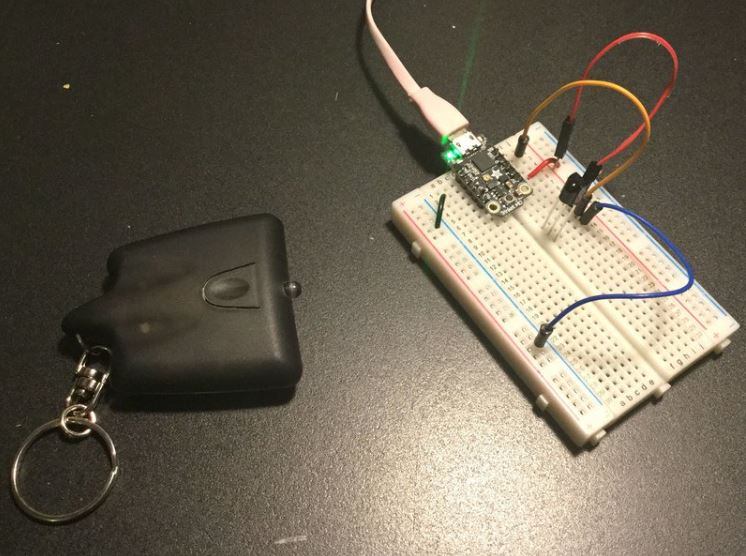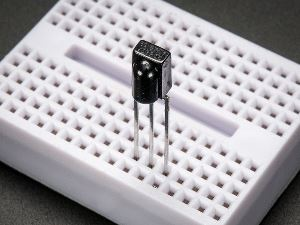IR (Infrared) Receiver Sensor

IR sensor tuned to 38KHz, perfect for receiving commands from a TV remote control. Runs at 3V to 5V so it's great for any microcontroller. To use, connect pin 3 (all the way to the...

Then on the Arduino or CircuitPython board, run a program that will capture the Infrared signals and print them out. We have some code on how to do that in this guide here. But then I realized right after I wired the whole thing up that it actually wouldn't work. Why? Because the IR receiver is tuned to 38KHz but the transmission bursts can be modulated at a bunch of different frequencies, sometimes as high as 56KHz. While the receiver will still likely receive the data, it could get garbled, and in any case, it's demodulated so we can't know the original encoding frequency, and the TV's we're blasting may be more sensitive.

It's Logic Analyzer Time!

OK no worries, we have the technology to solve this! Instead of using an IR receiver to demodulate the signal, we'll tap directly into the GPIO pin on the TV-B-Gone and listen to the signals. In this case we're going to use an old Saleae logic analyzer, but sigrok can also do the job and may be more affordable. The data rates we're talking about here, no faster than 60KHz, are fairly slow. It's just that the data is very long, the TV-B-Gone transmits for over a minute!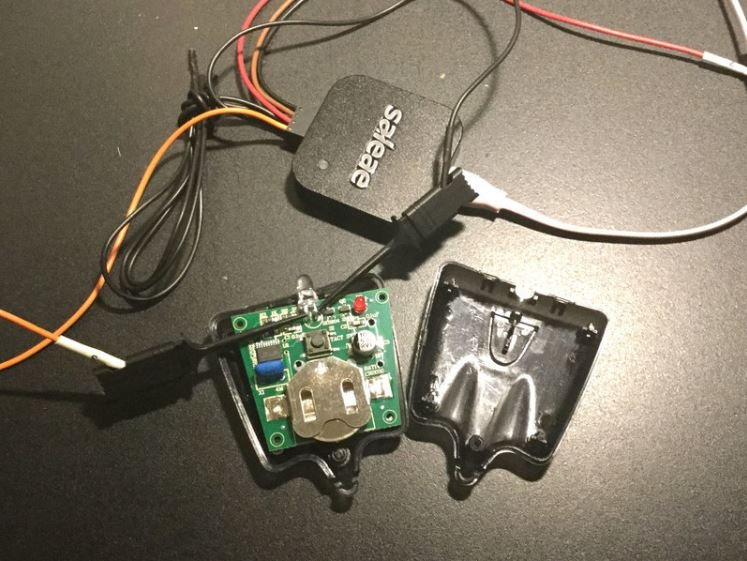Once we've performed the capture, we'll get a waveform like this: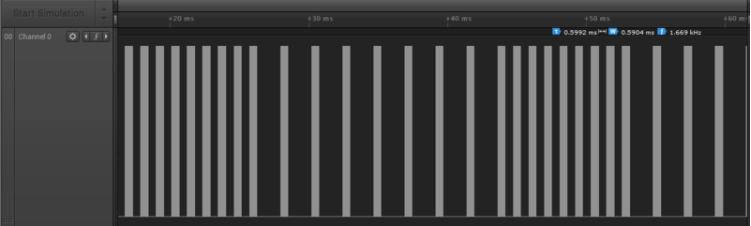Each of those 'pillars' is a pulse of infrared light at a certain frequency, the submodulation means it's easier for the TV receiver to tell the signal is for it. As mentioned before, that submodulation can vary, from 34KHz to 50KHz or more. If we zoom into the data we can see the details of each 'pillar' above: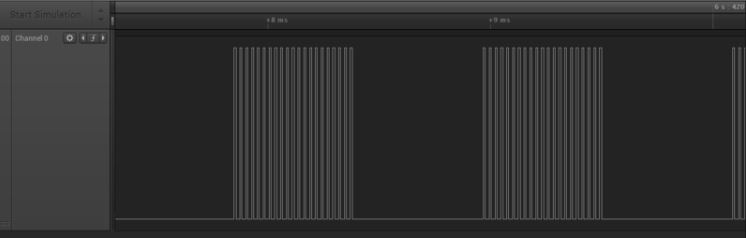Wonderful! now we just have to extract the frequencies, the time 'on' and the time 'off'. It's parsing time!

Parsing Data

Any logic analyzer with a software component will let you extract the data. Here's what the 'data dump' looks like from the Saleae software:

raw_tvbgone.csv

You can open up this data in any spreadsheet program, you'll see a long list with Sample column (sample time) and a Channel 0 (data) column. There's only one data column and we extracted only the transitions, so you'll see alternating numbers only.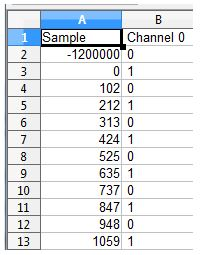The first sample is the pre-trigger (-1200000 us before trigger) you can just ignore that.

Afterwards, you see alternating 1's and 0's about 105 'somethings' apart. You might at first think it's maybe ms or microseconds, but it's not, it's actually the sample # based on the sample rate. You need to know that the rate we sampled at here is 12MHz so each sample point is 0.083 us. doing the math, the period between a 0, 1 and back to zero transition is ~210 samples. The period is 210 * 0.083us = 17.5us, which is the same as ~57KHz. So the first burst is 57KHz modulated.

We could go thru the entire 600,000 point CSV file but of course that would be tedious! Let's use python instead.

Jupyter to the Rescue

Our new favorite way to manage data with python is to use Jupyter (also referred to sometimes as a Python notebook) Jupyter is free, and lets you do data analysis with ease, I personally like that data is managed in chunks, so you can read in all the data in one chunk, then do math in other chunks, rather than re-running the whole thing over and over.

Here's our notebook, you can load it with any Jupyter install you've got

TV_B_Gone_parser.ipynb

Block #1

Let's start with the first block, where we read in the dataset: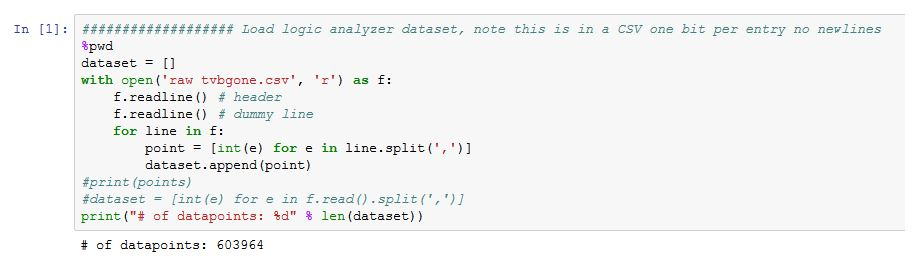Here, we open the 'raw tvbgone.csv' file as 'r'eadable text, then read the first two lines and toss them, then read each line, split the CSV into an list, then append the list to one big-ass list called dataset. At the end, we check, did we read the right number?Yep, last line is 603966 and we tossed the first line (text header) and first datapoint (the -1200000 pre-trigger marker) so 603964 is correct

Block #2

OK this block is where we do all the work. So we'll chunk it up into pieces.

In this code, we define our sample rate (12 MHz is a common rate), then loop thru the dataset, iterating through the length by 2's. the hi_p is 'high pulse', the amount of time we are at logic 1. lo_p is 'low pulse', the amount of time we are at logic 0. We also make a hi2_p which is the next pulse that goes high after the low pulse. We will fake this if we're at the end of the dataset, otherwise, we just take the next point.

Copy Code
`SAMPLERATE = 12000000       # 12 Mhz defaultunusual_codes =           # These are manchester coded or otherwise non-standard!frequency_pairs = pulse_points = # This function eats up two points at a time (but peeks at the third) # to calculate the high and low pulse lengths. As a pair, it determines# the frequency (usually 38KHz - 57KHz) and stores the freq in pulse_points# until it gets to a long low pulse (e.g. between bits or signals). It then# checks that the pulses so far are all the same frequency, and compresses # them into a triplet of the frequency, the amount of time that freq is emitted# and the amount of time the signal is 0 into frequency_pairsfor p in range(0, len(dataset), 2):  # take points two at a time    hi_p = datasetp    lo_p = datasetp+1    if (p+2) == len(dataset):        # we make a fake final pulse        hi2_p = lo_p[0 + 100000, 1]    else:        hi2_p = datasetp+2`

Now we do a quick assertion, that the high pulses should be value '1' and the low pulse should be value '0' and bail if somehow that happened.

Then, we take the actual length of time in samples of the high and low pulses, by taking the differences (deltas) between the pulse's timecode and the next one. Once we have the deltas, add them to make one cycle, and divide by the sample rate to convert to seconds, and then invert to get the frequency of those two pulses. This is basically the stuff we did by hand at the top of this page, but now it's done in code.

Copy Code
`        if (hi_p1 != 1) or (lo_p1 != 0) or (hi2_p1 != 1):        print("Error in matching pulse polarity")        exit(0)    delta_high = lo_p0 - hi_p0  # length of high pulse    delta_low = hi2_p0 - lo_p0  # length of low pulse    pulse_period = (delta_high + delta_low) / SAMPLERATE    pulse_freq = 1 / pulse_period    #print("%d, %d -> %0.2f" % (delta_high, delta_low, pulse_freq))  `

Now we've got a pulse of on/off light. Check at the bottom of this block and we have this section after our special-case checks;

# otherwise, add this pulse point

pulse_points.append(pulse_freq)

That is, assuming nothing special, we'll append the frequency reading we just made to a list for later handling. We'll do this 99% of the time, calculating the frequency of a pair of pulses, then appending until....

Now we come back to the special cases at the top of the if statement. If the low pulse is over 30 times longer than the high pulse, we're probably at the end of a pillar of modulated signal. (we picked 30 arbitrarily) Lets check if we have anything stored in pulse_points, if not it means we had a single blip of light, which is super weird (but did happen to us) So we store it in unusual_codes.

Otherwise, lets figure out what happened in this 'pillar' of pulses. We calculate avg_freq which is just the plain 'mean' average. Then we check that all the pulses are within 10% (over 0.9x and under 1.1x the average). If there is any such variation, we store for later and keep going. This did happen a few times, we just dropped these points.

Finally, if all the frequency-pulses in a pillar are within our exactly standards, we simplify them all down to a 3-part list. The list contains the average-frequency, the length that the pulses were active, and then that long-delta_low pulse converted to seconds.

We then loop around and keep going to the next 'pillar'

Copy Code
`if 30*delta_high < delta_low: # e.g. the last pulse        if not pulse_points:            print("#%d: %d, %d -> %0.2f" % (p, delta_high, delta_low, pulse_freq))            print("Found an unusual pulse, storing for later")            unusual_codes.append(p, delta_high, delta_low)            continue        # Lets get the avg frequency of all the pulse_points (they do have some slight variation)        avg_freq = sum(pulse_points) / len(pulse_points)        if not all( 0.9*avg_freq<i<1.1*avg_freq for i in pulse_points ):            print("#%d: %d, %d -> %0.2f" % (p, delta_high, delta_low, pulse_freq))            print("Found an unusual code, storing for later")            unusual_codes.append(pulse_points)            pulse_points =         # we'll just store the frequency, and the length of time on, then the length of time off        # We add one pulse for the 'final' pair we're on now        frequency_pairs.append(avg_freq, 1/avg_freq * (len(pulse_points)+1), delta_low / SAMPLERATE)        pulse_points =         continue # go to next pair of pulses# otherwise, add this pulse pointpulse_points.append(pulse_freq)`

Block #3

OK so far we've taken all the sub-modulated 1/0's and converted them to frequencies with on/off durations. In theory that's all we need to fully duplicate the TV-B-Gone, but it would be a huge amount of data and hard to manage. What we'll do now is group all the pulses within a chain, usually 10-30 are in a row, for an emitted code, and look like this (it's common to have one big burst in the beginning to 'get the attention' of the TV)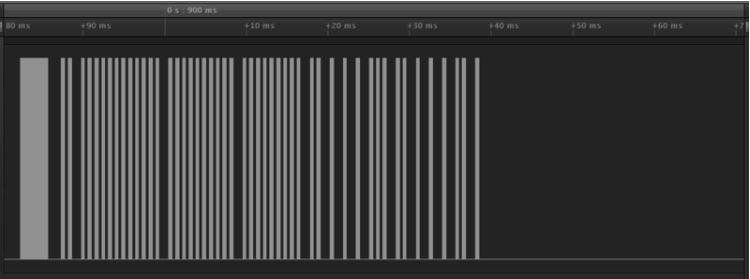In order to know when a code is done, we'll look back at the logic analyzer data. Just from scanning the data it seems like a lot of codes are 'repeated' about 65ms apart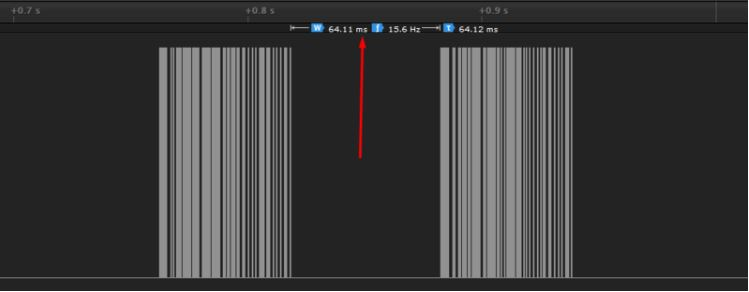And then there is a 0.25 second delay between code-types: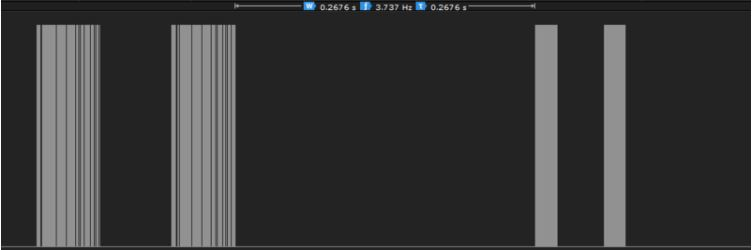We want to keep the 'duplicated' codes together (we'll deal with 'compressing' them later) so as a 'Intra Code Delay" we'll pick 0.2 seconds.

In block #3, we kind of do the same thing we did in block #2, but instead of individual light pulses, we'll group together modulated light chunks:

Copy Code
`# given the high frequency pairs, group them together by frequency and before a long (10ms?) pulseall_codes = code = INTRA_CODE_DELAY = 0.2   # in secondsfor f in frequency_pairs:    freq, high, low = f    #print("%0.2f %0.2f @ %0.1f" % (high * 1000, low * 1000, freq))    code.append(f)    if low > INTRA_CODE_DELAY:        code_freqs = p[0 for p in code]        avg_freq = sum(code_freqs) / len(code_freqs)        if not all( 0.9*avg_freq<i<1.1*avg_freq for i in code_freqs ):            print("Got an abberant frequency, bailing!")            code =             continue        only_pulses = [p[1, p2] for p in code]        all_codes.append({'freq':avg_freq, 'pulses':only_pulses})        code =         continueprint("Decoded: ", len(all_codes))`

For each on/off pair, we add it to our list called code. We keep going until the 'off' half of a pair is longer than that 0.2 seconds in which case we'll assume all the pairs till now are grouped together. We take the average modulation frequency of all the pairs and verify all are within 10%. Once we know they're all the same frequency, we don't have to save that part anymore, so only_pulse contains only the on/off timings. We then put those pulses in a dictionary that has the overall modulation frequency and the pulses, save it to all_codes and continue until we've finished processing all the on/off pairs.

According to our script, we've got 207 codes, which mean about 207 different brands/models of TVs.

If we ask Python to print out the first code with print(all_codes0) we'll get this: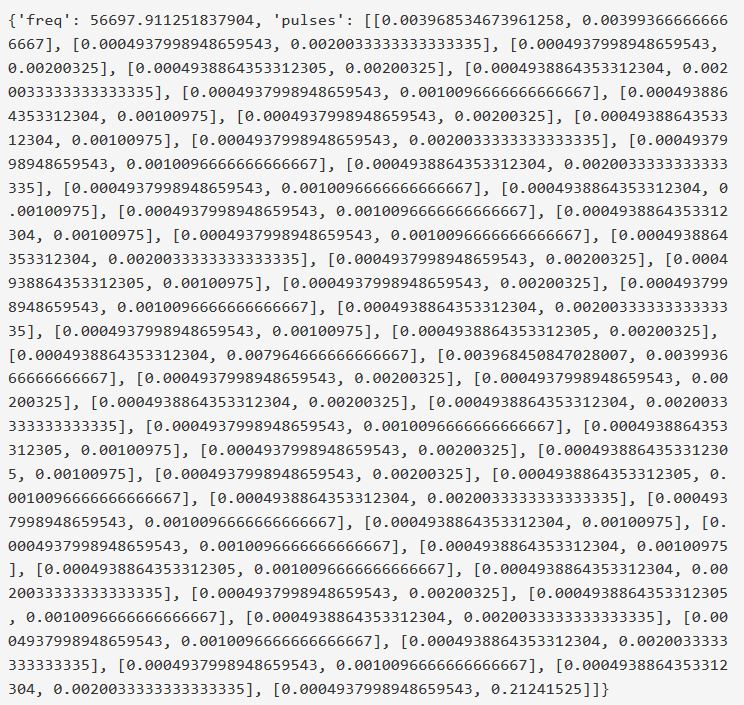Thanks to the precision of floating point numbers this is very wordy. Starting at the beginning, the dictionary item has {'freq': 56697.911251837904 which implies that the average frequency of this code is about 56.7KHz. If we look at the logic analyzer, we see that this is correct (each pulse has slight variation)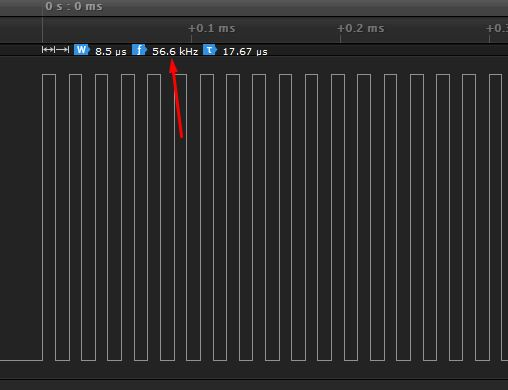Zooming out, the first pillar starts at 0ms and ends at about 4ms, then is off for about 4 ms. Then the next pillar of pulses starts at about 7.9ms and ends at 8.3ms (so about 0.4ms long).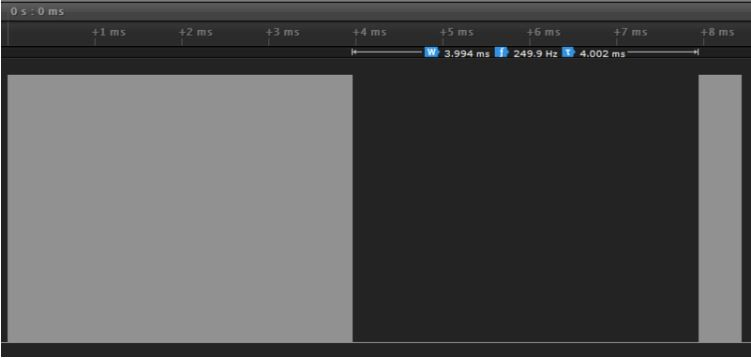That corresponds to the first few entries in our pulses list:

'pulses': [0.003968534673961258, 0.003993666666666667, 0.0004937998948659543, 0.0020033333333333335...

Note that all the times in the list are in seconds: Python has double-precision and we're not worried about running out of memory so doubles are fine for storage. Anyhow, it's always good to check what your parser puts out, compared to the raw data in the logic analyzer!

Let's continue!

Block #4

Now we've got all our codes in a nice dictionary format, with the frequency and on/off pulses stored away. We're going to keep making improvements to the formatting. Why? Well, for one, we want to compress the data a little so we can fit it on a Gemma. As is, the output of all_codes is 384KB

block3codes.txt

Which will work on a Circuit Playground Express or other Express boards. But we wanted to make it fit in a Gemma M0 for a super-compact project, and that would require the whole source code to take less than about 40KB. So, time for compression!

First up, those double-precision floats take up a lot more space ascii-wise than if we just converted to micro-seconds which will keep each entry at about 2-4 digits rather than the 6+ we have now:

Copy Code
`int_codes = for code in all_codes:    # convert to integers and make a dictionary    int_code = {'freq':int(code'freq')}    pulses =     for p in range(len(code'pulses')):        pulses.append(int(code'pulses'p0 * 1000000))   # convert to us        pulses.append(int(code'pulses'p1 * 1000000))   # convert to us    if len(pulses) % 2 == 0:        x = pulses.pop()        int_code'delay' = x / 1000000  # convert to s    int_code'pulses' = pulses`

We also make the dictionary object for the codes a little more comprehensive. To start, the frequency is converted to an integer (we really don't need to have more than 3 digits of precision for the frequency, so even this is overkill!) Then we go thru each pulse and multiply by 106. The very last entry, which is the final 'off' pulse, is removed, and renamed 'delay' and re-converted to seconds.

Next, remember we mentioned a lot of codes are repeated? That gives you a better chance of hitting the TV. So, the remainder of this block is dividing the pulses in half, then comparing each on/off timing entry to verify it's 'similar'

Copy Code
`    # lets see if we can cut it in half and compare both halves    half = len(int_code'pulses') // 2    left_half = int_code'pulses'0:half    repeat_delay = int_code'pulses'half    right_half = int_code'pulses'half+1:    #print(left_half)    #print(repeat_delay)    #print(right_half)    equiv = True    for i in range(len(left_half)):        if not similar(left_halfi, right_halfi):            equiv = False            break    if equiv:        # many/most codes repeat twice!        int_code'repeat' = 2        int_code'repeat_delay' = repeat_delay / 1000000 # convert to seconds        int_code'pulses' = left_half    else:        #print("NOT REPEAT!")        pass    int_codes.append(int_code)`

The middle 'off' pulse is the repeat delay, usually about 100ms (0.1 seconds). We have a helper function that checks if two values are within 5%, since the timings are slightly variant; we will accept that much variation to consider both 'halves' equivalent

Copy Code
`def similar(a, b, percent=0.05):  return (abs(1.0 - a / b) < percent)`

In theory we could check if the codes repeat 3 or 4 times instead of 2, but from scanning thru the data we could tell it was pretty much either once or twice per code.

Outputting all the int_codes, we see they now look like this (the first code)Which is way more compact than the previous floating point and non-repeat-optimized version. Our entire text file of codes is now 82 KB compared to the previous 382KB - a very nice compression that 'cost' us nothing.

block4codes.txt

Block #5

But....82KB is still too big, we need it to be less than half that. Let's look at more ways to compress the data. Looking at the first code: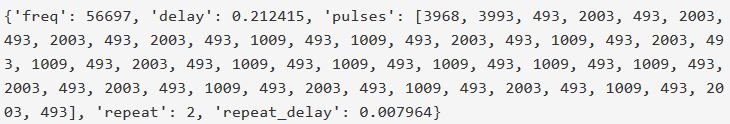We see some patterns. The numbers 493, 1009, and 2003 show up a lot. In fact, it's nearly all of the timing points! That's not too surprising, nearly all Infrared remotes send data that is encoded as 0's and 1's, and they do so with different length pulse pairs. In this code, there are 3 distinct pulse pairs:

1. 3968, 3993 - This is the initial 'attention' pulse, about 4000us on and 4000us off
2. 493, 2003 - about 500us on, 2000us off, will be decoded as a zero or one
3. 493, 1009 - about 500us on, 1000us off, will be decoded as the opposite as the above pulse

Instead of just repeating those full values over and over, lets 'compress' the pairs by just having a single digit number for each pair. That's what we'll do in the next block:

Copy Code
`paired_codes = for c in int_codes:    print('-'*40)    print(c)    pair_table =     pair_lookup =     for p in range(0, len(c'pulses'), 2):        pair = (c'pulses'p:p+2)        if len(pair) == 1:               # for the last entry, which is solitary            for pairs in pair_table:     # match it up with the first pair we find                if pair0 == pairs0:  # where the first pulse matches                    pair.append(pairs1)# (put in a false 'off' pulse)                    break        if not pair in pair_table:            pair_table.append(pair)                        pair_lookup.append(pair_table.index(pair))    p_code = {'freq': c'freq', 'delay': c'delay'}    try:        p_code'repeat' = c'repeat'        p_code'repeat_delay' = c'repeat_delay'    except KeyError:        pass    p_code'table' = pair_table    p_code'index' = pair_lookup    print(p_code)    paired_codes.append(p_code)`

After complete, you'll see comparisons of the pre-tableified and post codes like so: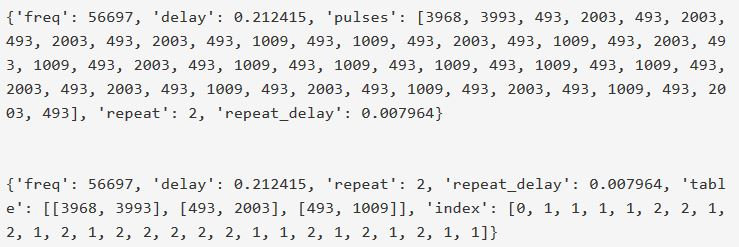As you can see, there's a new dictionary entry called 'table' with 3 entries: (3968, 3993), (493, 2003), (493, 1009) and then an index list, starting with a 0, then lots of 1's and 2's, those are the indicies into the pulse pair table.

Block #6

Now we're down to about 45KB - which is pretty good. We could try to convert all the codes into pure binary format instead of having indices, but considering the wide range of encoding schemes, and that we've essentially reached our target code size, we can stop.

We can squeeze just a tiny bit more space out by removing spaces and reducing the floating point precision. That's what the final block does, it rounds out the floating points and takes out all the whitespace, then writes the codes out to a text file that we can load into our CircuitPython Board

Copy Code
`# Compactify and print!with open("codes.txt", "w") as f:    for code in paired_codes:        code'delay' = round(code'delay',2)  # keep only 2 digits of precision for the long delay        try:            code'repeat_delay' = round(code'repeat_delay',3) # only 1ms precision for shot delay        except KeyError:            pass        s = str(code).replace(' ', '')  # remove whitespace!        print(s)        f.write(s+"\n")`

And here's the final output

codes.txt

The Magic of eval()

OK we've done the hard part; we've extracted all the data from a TV-B-Gone, captured, parsed and compressed it. Now we've got a 45KB file of TV power codes in the form of python 'dictionaries'.

This is where things get a little interesting, and we get to take advantage of CircuitPython being an interpreted language.

If you've written this kind of code before in C or C++, you'd think "OK lets compile the codes in as raw binary data or as structures, and then refer to them in the compiled code".

With CircuitPython, you might thus think "OK lets just paste the codes.txt file into main.py and then iterate over the list" But if you tried that you'd quickly realize that with codes.txt being 45KB and the ATSAMD21 only has 32KB of RAM, so it's impossible to load all this data into RAM.

Disk Storage

The next possible thought you might have is "ok well if I can't fit it all into RAM, lets put it on disk and parse the codes, we just have to write a parser" After all, that's how you'd do it with an Arduino C/C++

But, in python, we already have that parser! It's called eval() and it's the engine of how Python and CircuitPython works.

Let's look at eval for a moment, so you can see how handy it is!

Evaluating eval()

Open up your REPL to CircuitPython (we're using Mu) and type in the following:

Copy Code
`1+1`

You'll get this as a reply: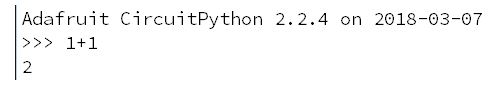2, is the evaluated reply to 1+1

If you think about it, the text you wrote "1+1" is basically a command - saying "Hey CircuitPython parser, please add two numbers, as I've given them to you". The "1+1" command is not compiled into code, there's no lookup table where "1+1" is an entry and "2" is the output. Instead, the CircuitPython interpreter must read that text, parse the text, realize that it's a valid Python, and then evaluate it, before printing the answer.

We can be somewhat flexible in how we give it the text. For example, it's valid Python to have a bunch of space between the + sign: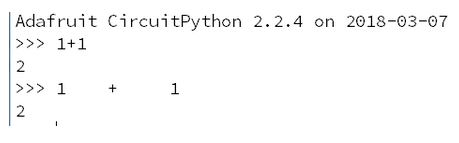But we cannot have spaces before the first digit: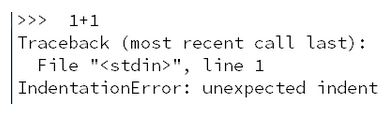Given that Python is interpreted, there is a function that takes the text you've typed in either here at the prompt, or in main.py and runs each line. That function is called eval, and you can run it by hand like so:

Copy Code
`eval("1+1")`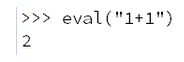Same as before, we get 2 back.

Since you're just passing in a character string, you can get quite creative. For example, you can create dynamic code like:

Copy Code
`import randommaths = "+", "-", "*", "/"eval("1" + mathsrandom.randint(0,3) + "2")`

This code creates a list with the 4 standard math operations, then evaluates a 'random' math operation between the integers 1 and 2 each time it's run - creating a different string to evaluate each time: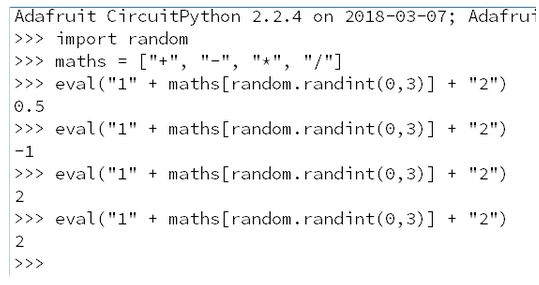Which, again, if you have only ever written compiled code, is very unusual.

But, since it's built into Python, we can take advantage of it to do parsing for us - we simply have CircuitPython read each line of code.txt which contains the printed out version of the dictionary item, and parse it directly as an evaluated string. Taa-dah, we've converted data on disk to code to data in memory with one function call!

CircuitPython Code

OK whew, finally we are ready to make our Circuit Playground Express zapper. There's no hardware changes or add-ons, you can use the CPX exactly as is thanks to the built-in IR emitter. You'll just need to save this code as main.py on your CPX:

Copy Code
`import boardimport arrayimport timefrom digitalio import DigitalInOut, Direction, Pullimport pulseio############## Switch to select 'stealth-mode'switch = DigitalInOut(board.SLIDE_SWITCH)switch.direction = Direction.INPUTswitch.pull = Pull.UP# Button to see output debugled = DigitalInOut(board.D13)led.direction = Direction.OUTPUT############## Speaker as haptic feedbackspkr_en = DigitalInOut(board.SPEAKER_ENABLE)spkr_en.direction = Direction.OUTPUTspkr_en.value = Truespkr = DigitalInOut(board.SPEAKER)spkr.direction = Direction.OUTPUT############## Allow any button to trigger activity!button_a = DigitalInOut(board.BUTTON_A)button_a.direction = Direction.INPUTbutton_a.pull = Pull.DOWNbutton_b = DigitalInOut(board.BUTTON_B)button_b.direction = Direction.INPUTbutton_b.pull = Pull.DOWNpwm = pulseio.PWMOut(board.REMOTEOUT, frequency=38000, duty_cycle=2 ** 15, variable_frequency=True)pulse = pulseio.PulseOut(pwm)while True:    # Wait for button press!    while not (button_a.value or button_b.value):        pass    time.sleep(0.5)  # Give a half second before starting        # gooooo!    f = open("/codes.txt", "r")    for line in f:        code = eval(line)        print(code)        pwm.frequency = code'freq'        if switch.value:            led.value = True        else:            spkr.value = True        # If this is a repeating code, extract details        try:            repeat = code'repeat'            delay = code'repeat_delay'        except KeyError:   # by default, repeat once only!            repeat = 1            delay = 0        # The table holds the on/off pairs        table = code'table'        pulses =   # store the pulses here        # Read through each indexed element        for i in code'index':            pulses += tablei  # and add to the list of pulses        pulses.pop()  # remove one final 'low' pulse        for i in range(repeat):            pulse.send(array.array('H', pulses))            time.sleep(delay)        led.value = False        spkr.value = False        time.sleep(code'delay')            f.close()`

And save this file as codes.txt on the CPX mini disk drive as well (it contains the 200+ IR codes)

codes.txt

Let's go through what this code actually does!

Stealth Mode

When you're in the field, you may want to avoid having something blinking in your hand. On the other hand, you want to know when it's done transmitting so you can move on to the next target. So we use the built in slide switch for 'Stealth Mode':

Copy Code
`############## Switch to select 'stealth-mode'switch = DigitalInOut(board.SLIDE_SWITCH)switch.direction = Direction.INPUTswitch.pull = Pull.UP# Button to see output debugled = DigitalInOut(board.D13)led.direction = Direction.OUTPUT############## Speaker as haptic feedbackspkr_en = DigitalInOut(board.SPEAKER_ENABLE)spkr_en.direction = Direction.OUTPUTspkr_en.value = Truespkr = DigitalInOut(board.SPEAKER)spkr.direction = Direction.OUTPUT`

If the switch is one way, the red LED will be used to blink when emitting a code. Flip the switch to have the speaker make a 'tic', a very small sound that you can hear if you're nearby but won't give you away!

Buttons and IR output

We'll use the two buttons to tell when it's time to zap! Since it's hard to remember which is which while out in the field, we set up both buttons:

Copy Code
`############## Allow any button to trigger activity!button_a = DigitalInOut(board.BUTTON_A)button_a.direction = Direction.INPUTbutton_a.pull = Pull.DOWNbutton_b = DigitalInOut(board.BUTTON_B)button_b.direction = Direction.INPUTbutton_b.pull = Pull.DOWN`

The built in REMOTEOUT pin is connected to the IR LED, we just create a PWM output on that pin. Even though we set up the frequency as 38000, we'll change the frequency for each code (thus the variable_frequency=True)

Copy Code
`pwm = pulseio.PWMOut(board.REMOTEOUT, frequency=38000, duty_cycle=2 ** 15, variable_frequency=True)pulse = pulseio.PulseOut(pwm)`

Main Loop

Now we're ready. In the main loop, we'll wait for any button press. If we get a press, we pause a moment (to get lined up) and then open that codes.txt file to read in IR codes!

Copy Code
`while True:    # Wait for button press!    while not (button_a.value or button_b.value):        pass    time.sleep(0.5)  # Give a half second before starting        # gooooo!    f = open("/codes.txt", "r")`

Each line contains that dictionary entry. We use the magical eval() function to convert the text to a Python object. Depending on the switch position we either turn on the LED or give the speaker a pulse high.

Copy Code
`for line in f:        code = eval(line)        print(code)        if switch.value:            led.value = True        else:            spkr.value = True`

Then we can check the dictionary. Repeating codes have a 'repeat' and 'repeat_delay' entry. If not, we'll assume we're transmitting only once.

Copy Code
`        # If this is a repeating code, extract details        try:            repeat = code'repeat'            delay = code'repeat_delay'        except KeyError:   # by default, repeat once only!            repeat = 1            delay = 0`

Then, we can take that code-pair table out, and 'de-index' the table to recreate the original on/off list (we need that list for the pulse output send function)

Copy Code
`        # The table holds the on/off pairs        table = code'table'        pulses =   # store the pulses here        # Read through each indexed element        for i in code'index':            pulses += tablei  # and add to the list of pulses        pulses.pop()  # remove one final 'low' pulse`

Finally, set the PWM output frequency to whatever the TV is listening for, and send the pulse on/off codes, repeating if desired.

Once done, turn off the LED and speaker, and have one long inter-code delay.

Copy Code
`        pwm.frequency = code'freq'        for i in range(repeat):            pulse.send(array.array('H', pulses))            time.sleep(delay)                led.value = False        spkr.value = False        time.sleep(code'delay')`

And when done, close the file so we can start over, waiting for another button press

Copy Code
`    f.close()`

That's it! You may want to run the code on the REPL the first time, to make sure you've got everything set up.

Note that the IR LED draws a couple hundred milli-Amps when sending IR data, so a good battery pack will help you get that range. You can expect up to 30 feet, depending on your targeting skills!

Gemma M0 Variant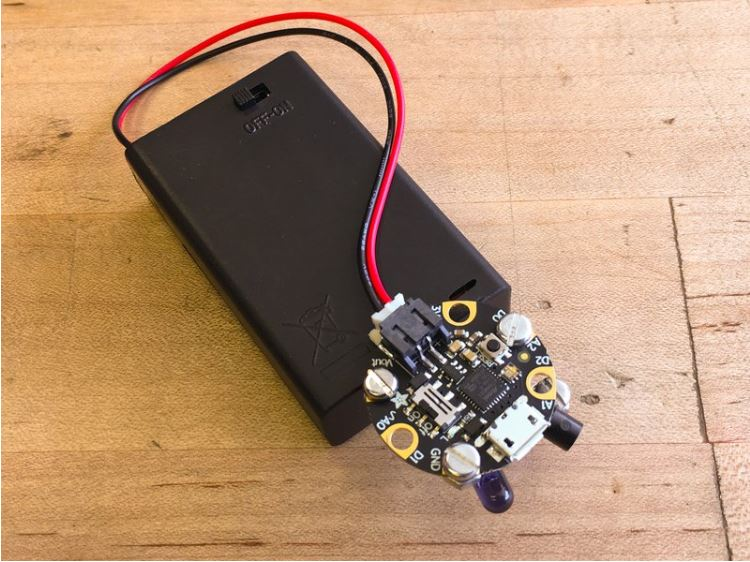You may be thinking, "Hey, this TV B GONE looks awesome, but I have a Gemma M0, can I get in on the fun?" Yes you can!

Here's what you'll need to build it:1 x Gemma M0 Little round microcontroller

1 x IR LED Super bright, 5mm 940nm

1 x Battery Holder 3x AAA with On/Off switch

1 x Alkaline Batteries 3x AAA

1 x 2N7000 Transistor

4 x M3 x 8mm Screws and Nuts

Build the Gemma M0 TV B GONE

We could connect the IR LED directly to the Gemma M0, but it would have a pretty close range -- in order to boost the power, we'll use a transistor. The transistor will act as an amplifier to boost the current that flows through the LED.

Here's the circuit diagram we'll use.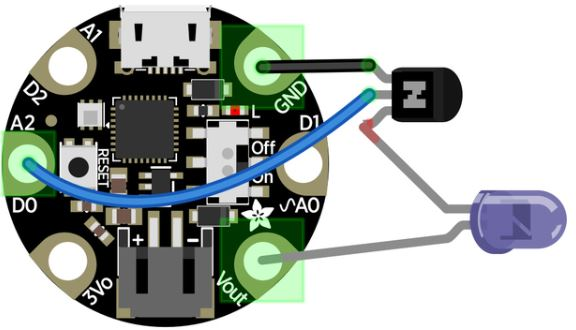We'll make all of the connections using M3 x 8mm screws and nuts.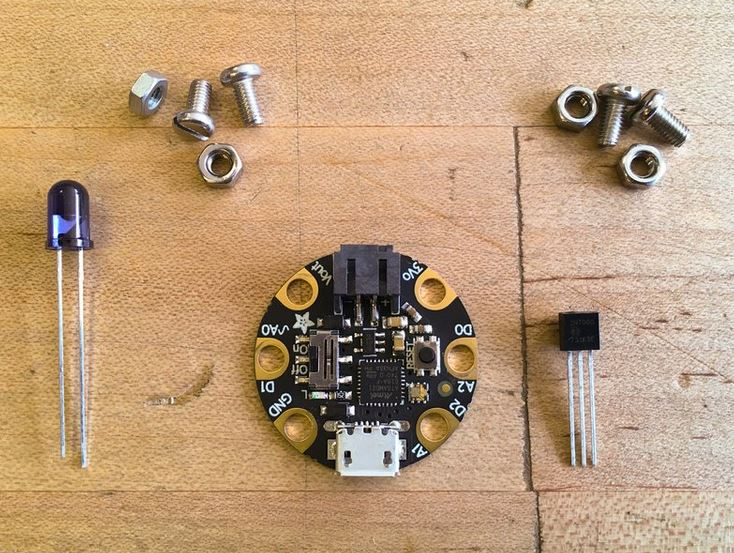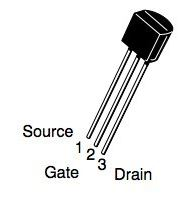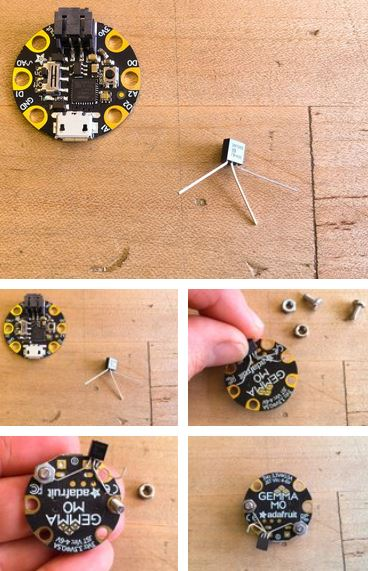• First, take the transistor and carefully spread the legs as shown
• Bend slightly hooked feet on the source and gate legs
• Screw the source leg in place to GND and the gate leg to A0 as shown
• Note how the drain leg is bent up a bit to connect later to the cathode leg of the IR LED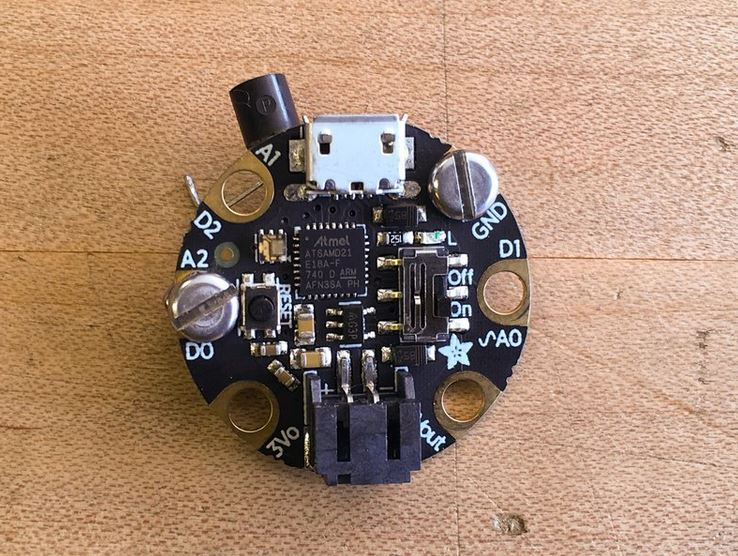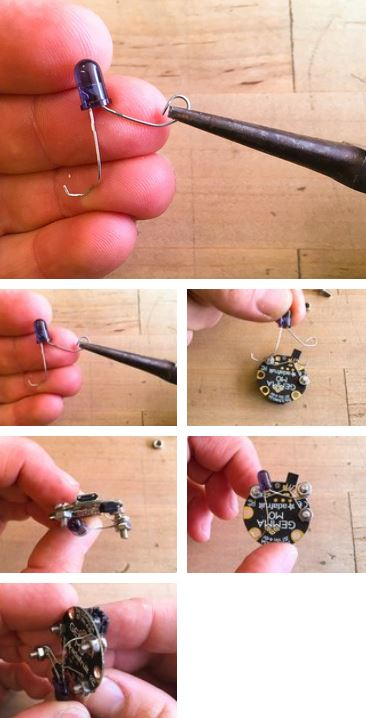• Next, bend hooks at the ends of both LED legs so they can be connected
• Connect the shorter cathode leg with a screw and nut to the drain leg of the transistor as shown
• Then, connect the longer anode leg connect to the Gemma M0's A0 pad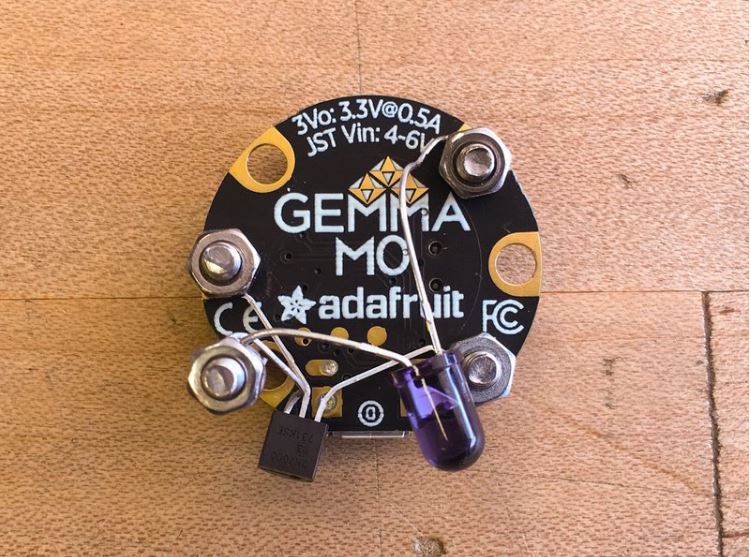Power

The Gemma M0 TV B GONE needs power! You can plug in your AAA battery pack, and even affix the Gemma M0 to the case with some double sided foam tape.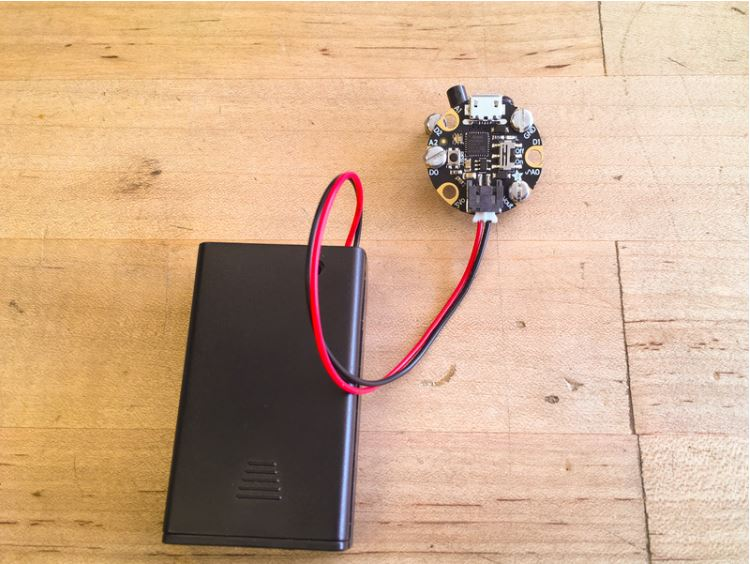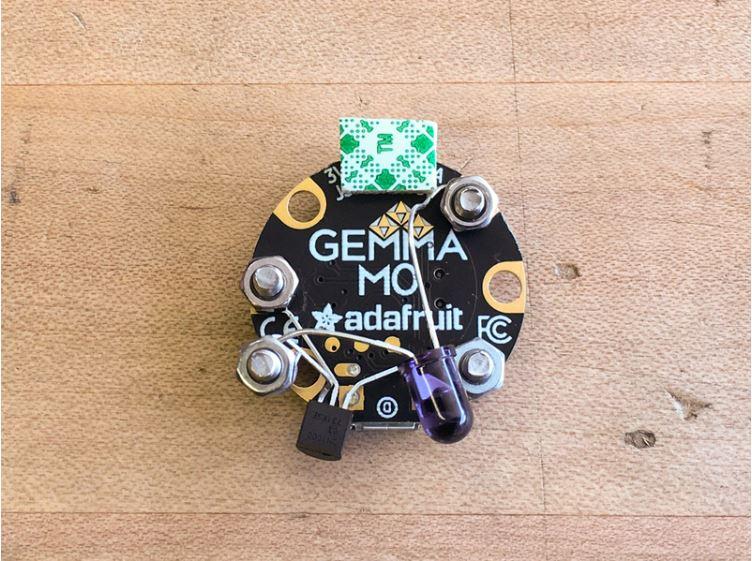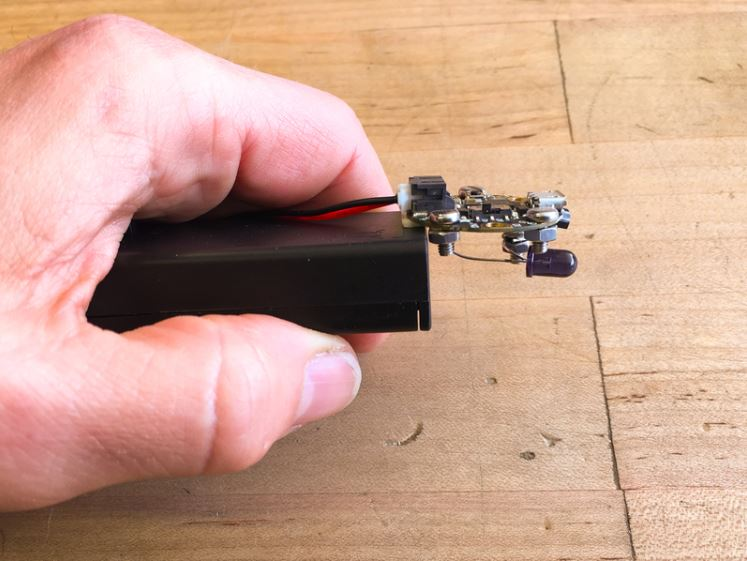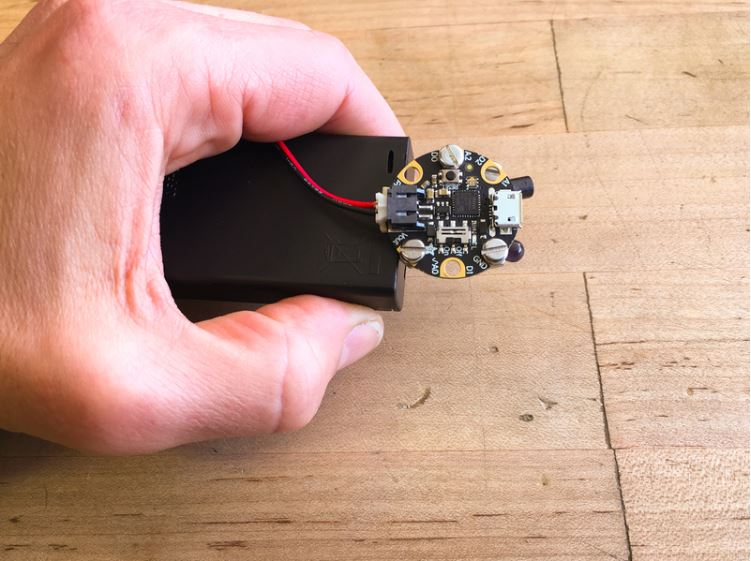Coding with CircuitPython

We'll code the Gemma M0 using CircuitPython. First, follow this guide https://learn.adafruit.com/adafruit-gemma-m0/circuitpython to get started with coding the Gemma M0 in CircuitPython. Install the latest release version of CircuitPython on the board.

You may also want to install the Mu editor https://learn.adafruit.com/adafruit-gemma-m0/installing-mu-editor for your coding needs.

Once you can successfully code in Mu and upload to the board, return here.

The program we'll use is quite small, but we do need to provide a large list of TV off codes as a separate text file on the Gemma M0. In order to save some space, we'll delete some unneeded things from the Gemma M0's CIRCUITPY drive: you can trash the Windows 7 Driver folder, as well as two items from the lib folder: adafruit_hid and neopixel.mpy.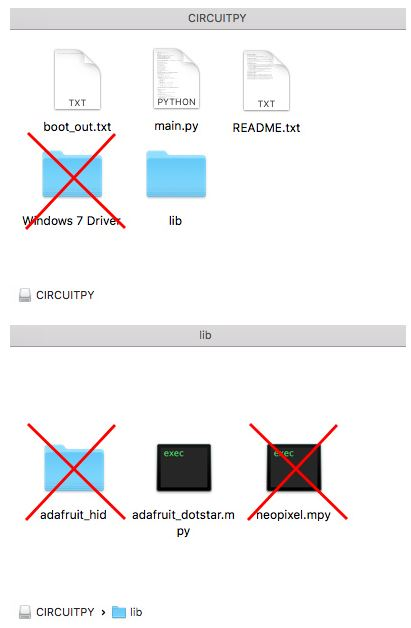Now, that there's enough space, download the codes file here as codes.txt onto the root of the CIRCUITPY drive.

codes.txt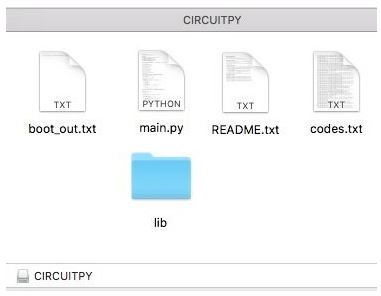This is the code for the Gemma M0 TV B GONE. Copy it and then paste it into Mu. Save the file as main.py onto your Gemma M0's CIRCUITPY drive (you can replace the existing main.py file) and it's ready to go!

Copy Code
`# Gemma M0 version of TVBgone!import boardimport arrayimport timefrom digitalio import DigitalInOut, Direction, Pullimport pulseioimport adafruit_dotstarpixel = adafruit_dotstar.DotStar(board.APA102_SCK, board.APA102_MOSI, 1, brightness=0.2)pixel.fill((0, 0, 0))# Button to see output debugled = DigitalInOut(board.D13)led.direction = Direction.OUTPUTpwm = pulseio.PWMOut(board.A1, frequency=38000, duty_cycle=2 ** 15, variable_frequency=True)pulse = pulseio.PulseOut(pwm)time.sleep(0.5)  # Give a half second before starting    # gooooo!f = open("/codes.txt", "r")for line in f:    code = eval(line)    print(code)    pwm.frequency = code'freq'    led.value = True    # If this is a repeating code, extract details    try:        repeat = code'repeat'        delay = code'repeat_delay'    except KeyError:   # by default, repeat once only!        repeat = 1        delay = 0    # The table holds the on/off pairs    table = code'table'    pulses =   # store the pulses here    # Read through each indexed element    for i in code'index':        pulses += tablei  # and add to the list of pulses    pulses.pop()  # remove one final 'low' pulse    for i in range(repeat):        pulse.send(array.array('H', pulses))        time.sleep(delay)    led.value = False    time.sleep(code'delay')    f.close()`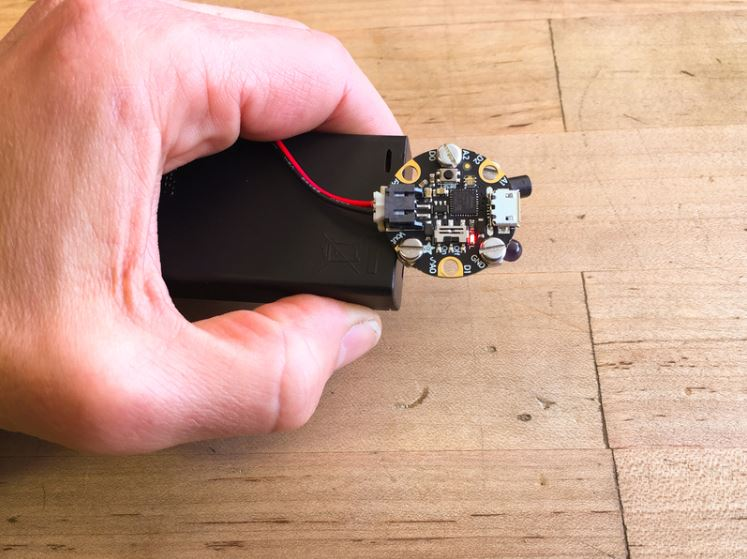It's ready for action! Turn it on and watch the TVs go dark!!

You'll see the onboard red LED blink every time a TV code is sent. It can take a sometime for it to run through every code in the list, but the time it's done, there's a very good chance it has set some TV sets to sleep!

## Key Parts and Components

Add all Digi-Key Parts to Cart
• 1528-2348-ND
• 1528-1151-ND
• 1528-2280-ND
• 1528-2282-ND
• 1528-1655-ND
• 751-1227-ND